# Light and Reflection Light Characteristics of Light Not

• Slides: 39Light and Reflection Light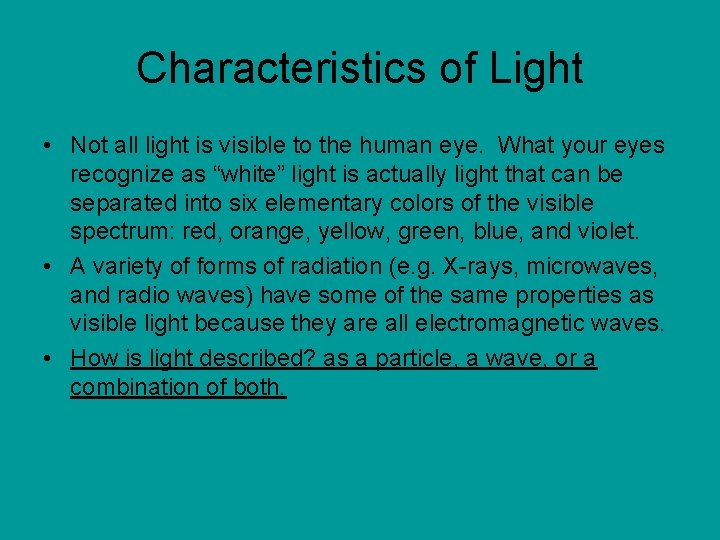Characteristics of Light • Not all light is visible to the human eye. What your eyes recognize as “white” light is actually light that can be separated into six elementary colors of the visible spectrum: red, orange, yellow, green, blue, and violet. • A variety of forms of radiation (e. g. X-rays, microwaves, and radio waves) have some of the same properties as visible light because they are all electromagnetic waves. • How is light described? as a particle, a wave, or a combination of both.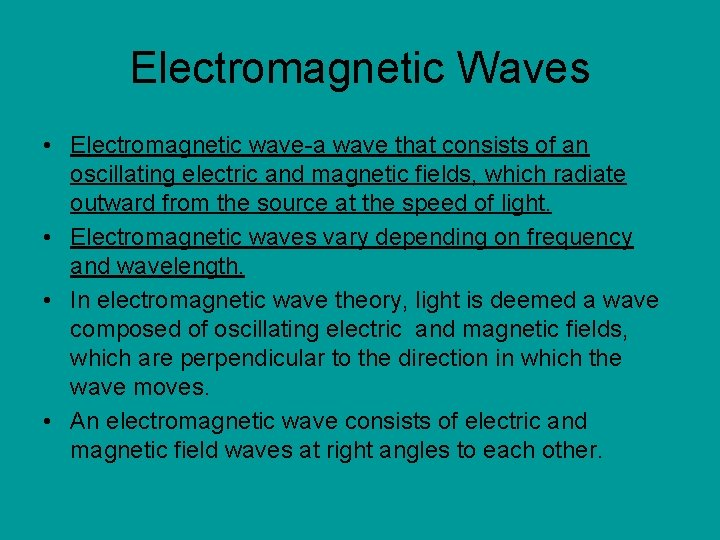Electromagnetic Waves • Electromagnetic wave-a wave that consists of an oscillating electric and magnetic fields, which radiate outward from the source at the speed of light. • Electromagnetic waves vary depending on frequency and wavelength. • In electromagnetic wave theory, light is deemed a wave composed of oscillating electric and magnetic fields, which are perpendicular to the direction in which the wave moves. • An electromagnetic wave consists of electric and magnetic field waves at right angles to each other.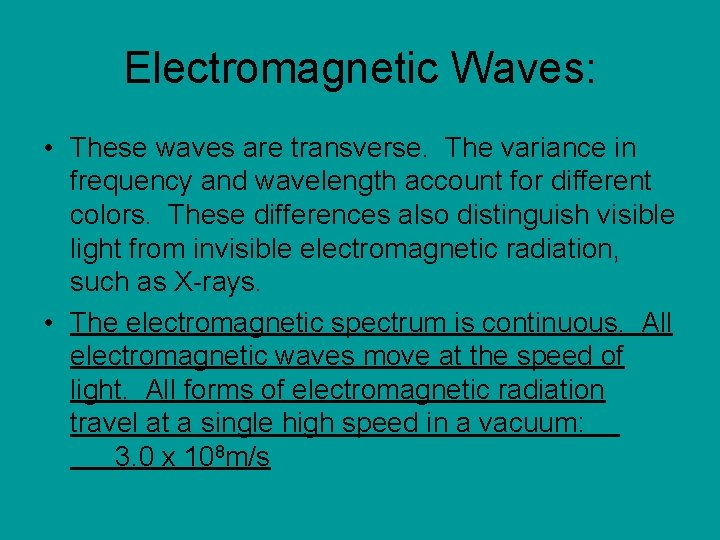Electromagnetic Waves: • These waves are transverse. The variance in frequency and wavelength account for different colors. These differences also distinguish visible light from invisible electromagnetic radiation, such as X-rays. • The electromagnetic spectrum is continuous. All electromagnetic waves move at the speed of light. All forms of electromagnetic radiation travel at a single high speed in a vacuum: 3. 0 x 108 m/sWave Speed: c=fλ, c (speed of light). • Sample Problem A: The AM Radio band extends from 5. 4 x 105 Hz to 1. 7 x 106 Hz. What are the longest and shortest wavelengths in this frequency range?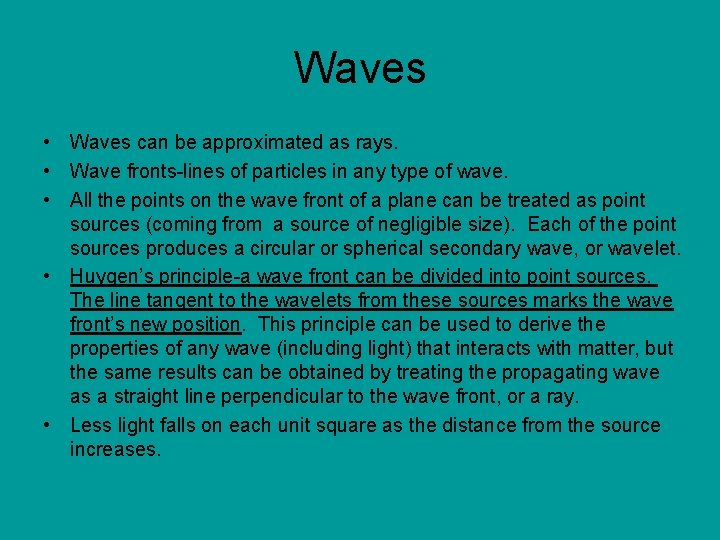Waves • Waves can be approximated as rays. • Wave fronts-lines of particles in any type of wave. • All the points on the wave front of a plane can be treated as point sources (coming from a source of negligible size). Each of the point sources produces a circular or spherical secondary wave, or wavelet. • Huygen’s principle-a wave front can be divided into point sources. The line tangent to the wavelets from these sources marks the wave front’s new position. This principle can be used to derive the properties of any wave (including light) that interacts with matter, but the same results can be obtained by treating the propagating wave as a straight line perpendicular to the wave front, or a ray. • Less light falls on each unit square as the distance from the source increases.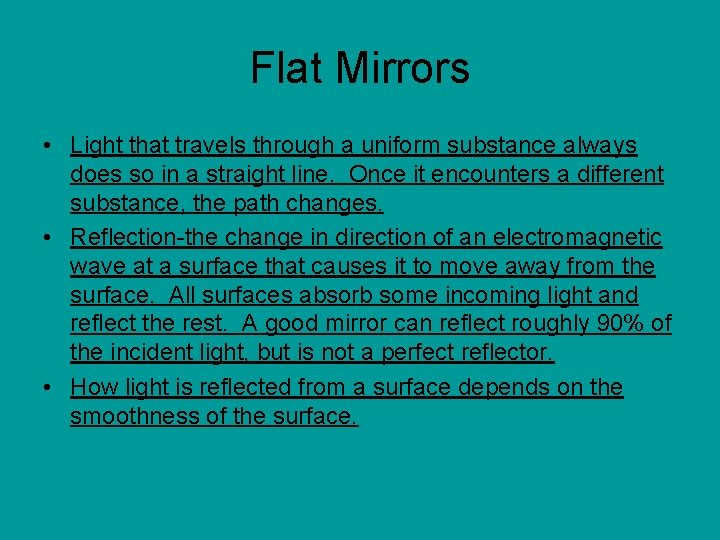Flat Mirrors • Light that travels through a uniform substance always does so in a straight line. Once it encounters a different substance, the path changes. • Reflection-the change in direction of an electromagnetic wave at a surface that causes it to move away from the surface. All surfaces absorb some incoming light and reflect the rest. A good mirror can reflect roughly 90% of the incident light, but is not a perfect reflector. • How light is reflected from a surface depends on the smoothness of the surface.Flat Mirrors • Diffuse reflection occurs when light is reflected from a rough, textured surface (e. g. cloth). • Specular reflection occurs with light reflection from smooth, shiny surfaces (e. g. mirror). • Specular-one direction only, Diffuse-many directions. • A surface is considered smooth if its surface variations are small compared with the wavelength of the incoming light. Incoming and reflected angles are equal.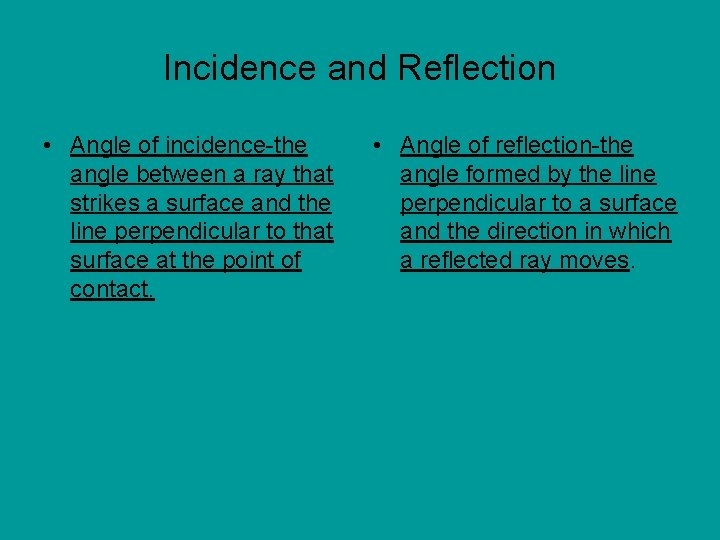Incidence and Reflection • Angle of incidence-the angle between a ray that strikes a surface and the line perpendicular to that surface at the point of contact. • Angle of reflection-the angle formed by the line perpendicular to a surface and the direction in which a reflected ray moves.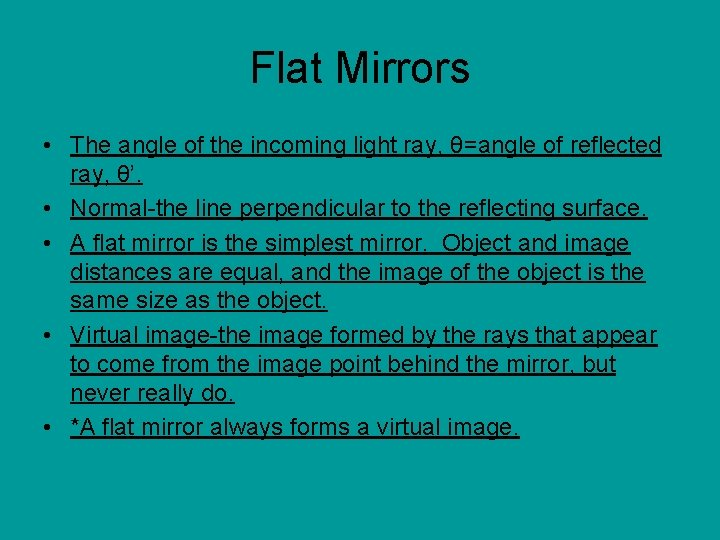Flat Mirrors • The angle of the incoming light ray, θ=angle of reflected ray, θ’. • Normal-the line perpendicular to the reflecting surface. • A flat mirror is the simplest mirror. Object and image distances are equal, and the image of the object is the same size as the object. • Virtual image-the image formed by the rays that appear to come from the image point behind the mirror, but never really do. • *A flat mirror always forms a virtual image.Curved Mirrors • Small circular mirrors, such as those on dressing tables may appear to be the same as flat mirrors. However, they form different images. Images for objects closer to the mirror are larger than those which reside farther from the mirror which are smaller and upside down. These are curved mirrors. The larger image is a virtual one, and the smaller one is real. Concave mirrors can be used to form real images. Spherical mirrors are spherical in shape. • Concave spherical mirror-a mirror whose reflecting surface is a segment of the inside of a sphere.When are concave mirrors used? • Whenever a magnified image of an object is needed. (e. g. a dressing mirror). • Radius of curvature is a factor that determines where the image will appear in a concave spherical mirror, and how large it will be. • R=distance from surface of mirror to the center of curvature, C.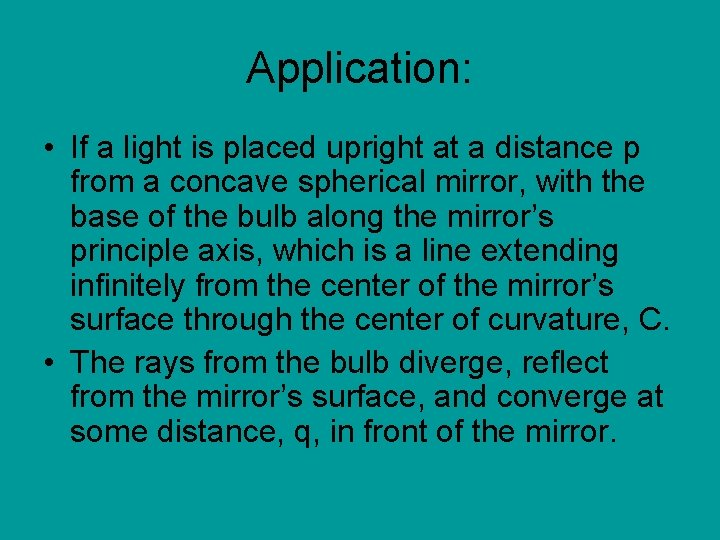Application: • If a light is placed upright at a distance p from a concave spherical mirror, with the base of the bulb along the mirror’s principle axis, which is a line extending infinitely from the center of the mirror’s surface through the center of curvature, C. • The rays from the bulb diverge, reflect from the mirror’s surface, and converge at some distance, q, in front of the mirror.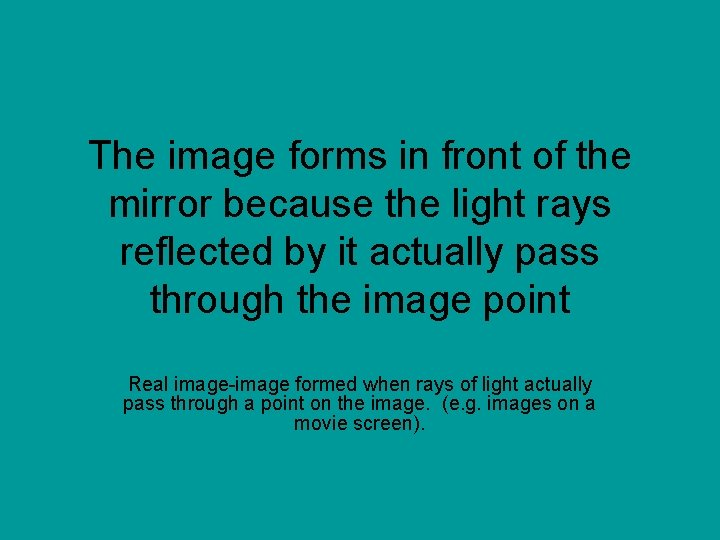The image forms in front of the mirror because the light rays reflected by it actually pass through the image point Real image-image formed when rays of light actually pass through a point on the image. (e. g. images on a movie screen).Real Images • Real images can be displayed on a surface unlike the virtual images that appear behind a flat mirror. Why? Because they appear in front. • An image created by spherical mirror sufferers from spherical aberration. In this situation, certain rays don’t exactly intersect at the image point. • Paraxial rays-those light rays that are very near the principle axis of the mirror. • Image location can be predicted with the mirror equation.Images and Mirrors • Object distance, p, image distance q, and radius of curvature, R, are interdependent. • How can you predict where an image will appear? You need to know the object distance and radius of curvature. • If a light bulb is placed far from a mirror, the object distance is great enough compared with R that 1/p is almost zero, and q is almost R/2, so the image would form from about halfway between the center of curvature and the center of the mirror’s surface. The image point is the focal point of the mirror, F. Image distance is focal length, f.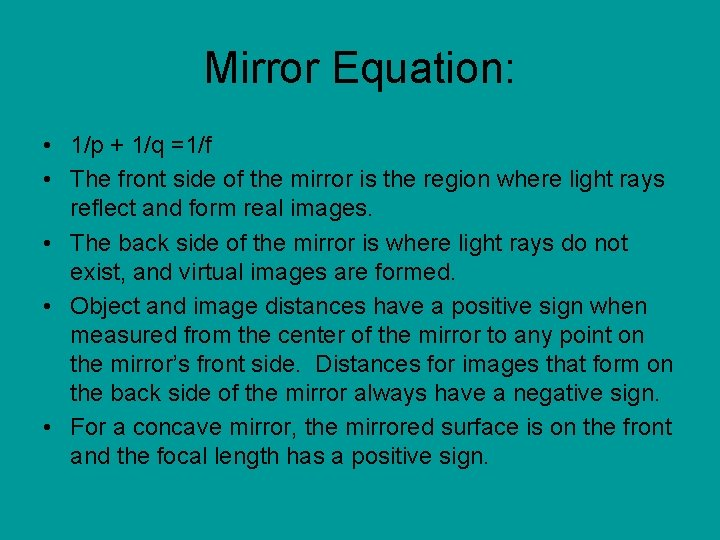Mirror Equation: • 1/p + 1/q =1/f • The front side of the mirror is the region where light rays reflect and form real images. • The back side of the mirror is where light rays do not exist, and virtual images are formed. • Object and image distances have a positive sign when measured from the center of the mirror to any point on the mirror’s front side. Distances for images that form on the back side of the mirror always have a negative sign. • For a concave mirror, the mirrored surface is on the front and the focal length has a positive sign.Mirrors • The object and image height are positive when both are above the principal axis and negative when either is below. • Magnification relates image and object sizes. • Unlike flat mirrors, curved mirrors form images that are not the same size as the object. • Magnification is the measure of how large or small the image is with respect to the original object’s size; the ratio of the height of the bulb’s image to the bulb’s actual height. M also equals the negative of the ratio of the image distance to the object distance. • *If an image is smaller than the object, the magnitude of magnification is less than 1, and vice versa. Magnification has no units. • M=h’/h=-q/p, image height/object height=image distance/object distance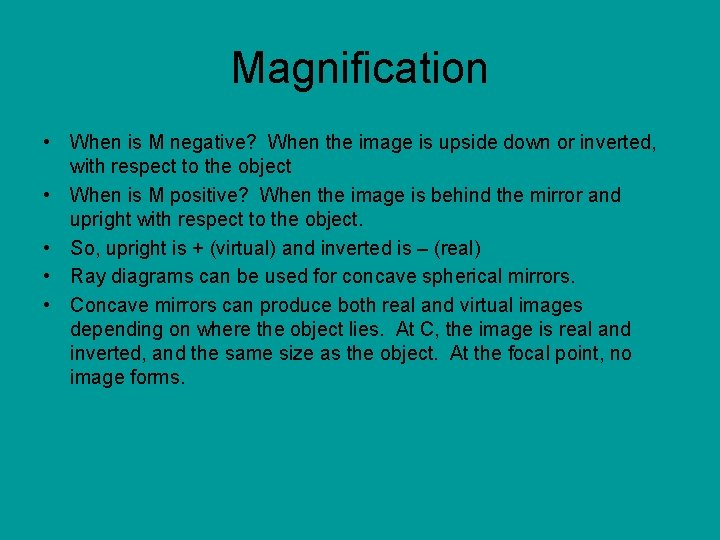Magnification • When is M negative? When the image is upside down or inverted, with respect to the object • When is M positive? When the image is behind the mirror and upright with respect to the object. • So, upright is + (virtual) and inverted is – (real) • Ray diagrams can be used for concave spherical mirrors. • Concave mirrors can produce both real and virtual images depending on where the object lies. At C, the image is real and inverted, and the same size as the object. At the focal point, no image forms.Sample Problem B • A concave spherical mirror has a focal length of 10. 0 -cm. Locate the image of a pencil that is placed upright 30. 0 -cm from the mirror. Find the magnification of the image.Convex Spherical Mirrors • Convex spherical mirror-a mirror whose reflecting surface is an outward-curved segment of a sphere. Ex. Side view mirror passenger’s side of the car. This is diverging because the incoming rays diverge after reflection as though they were coming from some point behind the mirror. These are mirrors placed in stores to monitor customer shopping.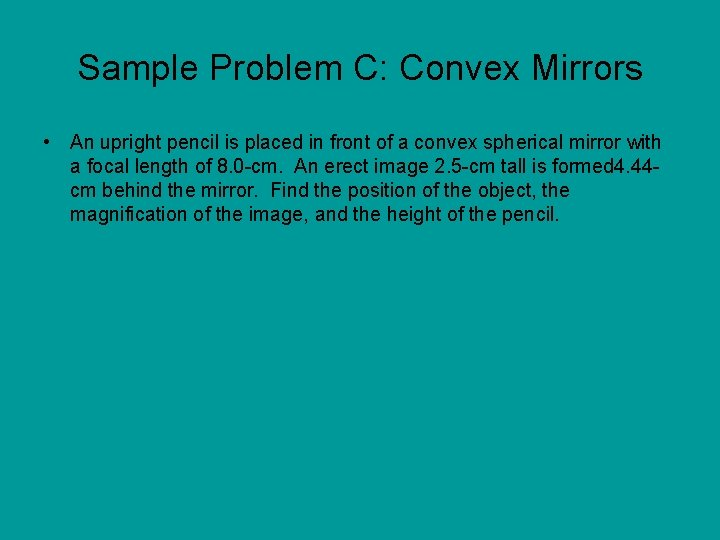Sample Problem C: Convex Mirrors • An upright pencil is placed in front of a convex spherical mirror with a focal length of 8. 0 -cm. An erect image 2. 5 -cm tall is formed 4. 44 cm behind the mirror. Find the position of the object, the magnification of the image, and the height of the pencil.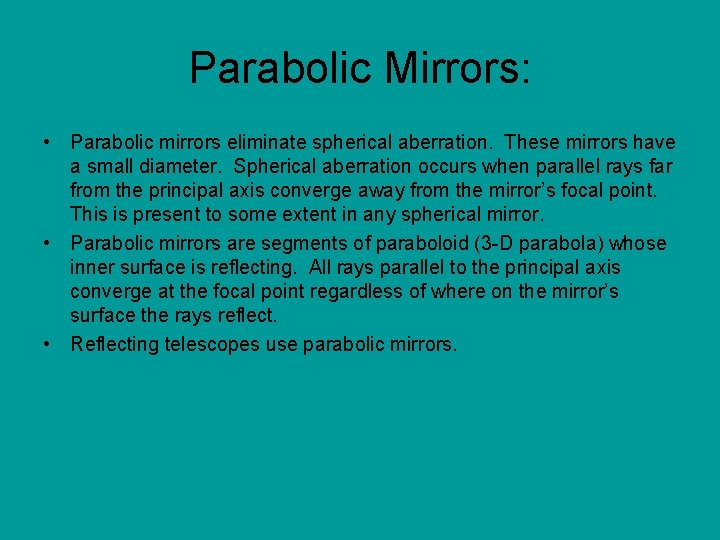Parabolic Mirrors: • Parabolic mirrors eliminate spherical aberration. These mirrors have a small diameter. Spherical aberration occurs when parallel rays far from the principal axis converge away from the mirror’s focal point. This is present to some extent in any spherical mirror. • Parabolic mirrors are segments of paraboloid (3 -D parabola) whose inner surface is reflecting. All rays parallel to the principal axis converge at the focal point regardless of where on the mirror’s surface the rays reflect. • Reflecting telescopes use parabolic mirrors.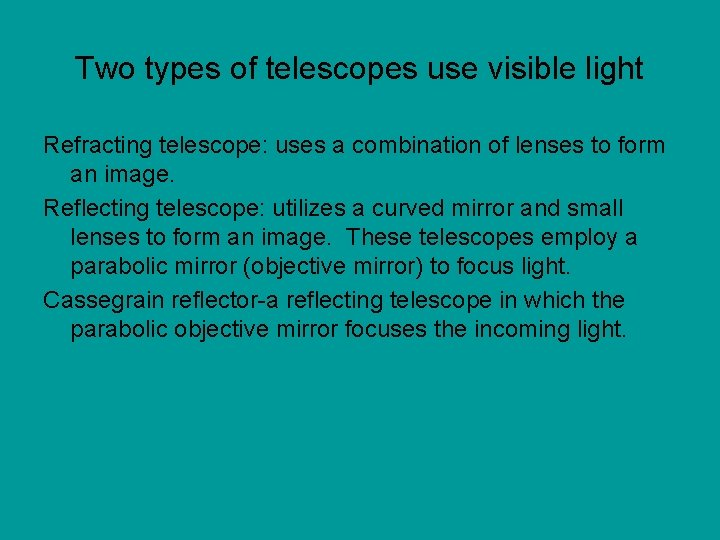Two types of telescopes use visible light Refracting telescope: uses a combination of lenses to form an image. Reflecting telescope: utilizes a curved mirror and small lenses to form an image. These telescopes employ a parabolic mirror (objective mirror) to focus light. Cassegrain reflector-a reflecting telescope in which the parabolic objective mirror focuses the incoming light.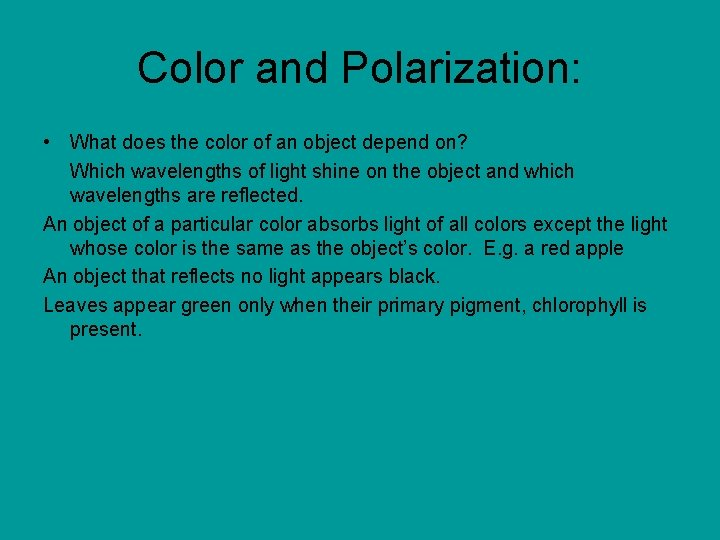Color and Polarization: • What does the color of an object depend on? Which wavelengths of light shine on the object and which wavelengths are reflected. An object of a particular color absorbs light of all colors except the light whose color is the same as the object’s color. E. g. a red apple An object that reflects no light appears black. Leaves appear green only when their primary pigment, chlorophyll is present.Primary colors • Red • Green • Blue • *The primary combinations produce yellow, pink, and white.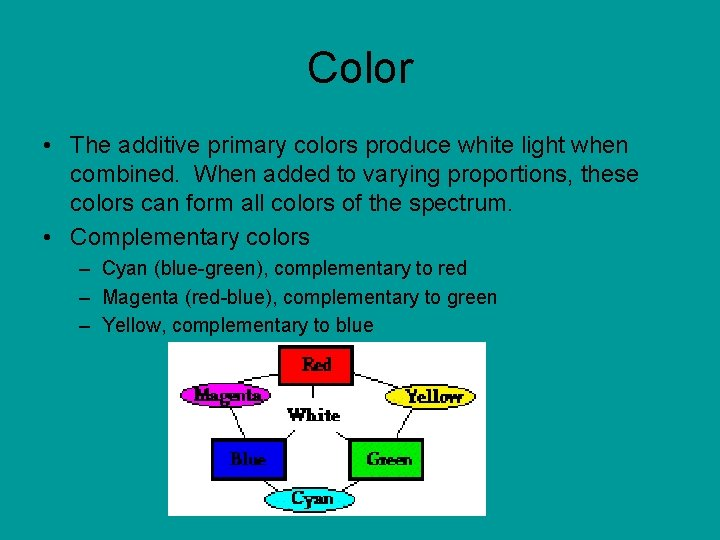Color • The additive primary colors produce white light when combined. When added to varying proportions, these colors can form all colors of the spectrum. • Complementary colors – Cyan (blue-green), complementary to red – Magenta (red-blue), complementary to green – Yellow, complementary to blue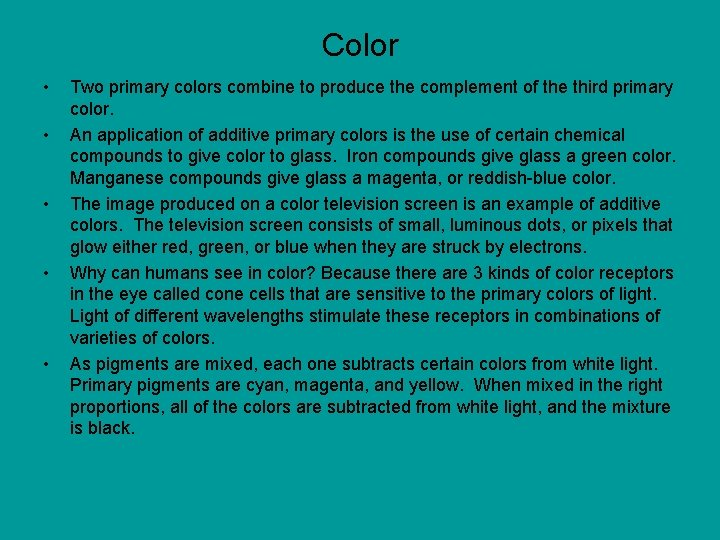Color • • • Two primary colors combine to produce the complement of the third primary color. An application of additive primary colors is the use of certain chemical compounds to give color to glass. Iron compounds give glass a green color. Manganese compounds give glass a magenta, or reddish-blue color. The image produced on a color television screen is an example of additive colors. The television screen consists of small, luminous dots, or pixels that glow either red, green, or blue when they are struck by electrons. Why can humans see in color? Because there are 3 kinds of color receptors in the eye called cone cells that are sensitive to the primary colors of light. Light of different wavelengths stimulate these receptors in combinations of varieties of colors. As pigments are mixed, each one subtracts certain colors from white light. Primary pigments are cyan, magenta, and yellow. When mixed in the right proportions, all of the colors are subtracted from white light, and the mixture is black.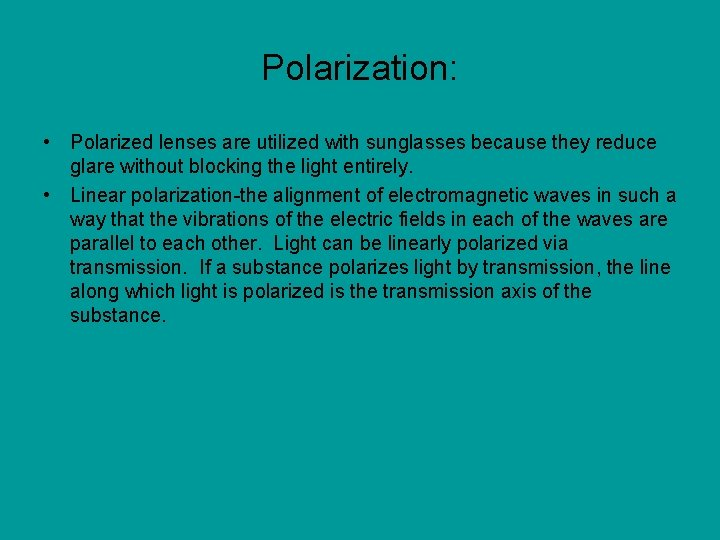Polarization: • Polarized lenses are utilized with sunglasses because they reduce glare without blocking the light entirely. • Linear polarization-the alignment of electromagnetic waves in such a way that the vibrations of the electric fields in each of the waves are parallel to each other. Light can be linearly polarized via transmission. If a substance polarizes light by transmission, the line along which light is polarized is the transmission axis of the substance.Refraction • • Refraction-the bending of light as it travels from one medium to another. The normal is the line extending into the refracting medium. Angle of refraction, θr-the angle between the refracted ray and the normal. When does refraction occur? When light’s velocity changes. What types of transparent media does light pass through? Glass, water, ice, and diamonds, and quartz. The speed of light in each of these materials is different. The speed of light in water is less than the speed of light in air. As light moves from one medium to another, part of it is reflected and part is refracted. As a light ray travels from air into glass, the refracted portion is bent toward the normal, however, the path of the light ray moving from glass into air is bent away from the normal. If the incident ray of light is parallel to the normal, then no refraction (bend) occurs in either case. Refraction can be explained via the wave model of light using wave fonts and light rays. Refraction occurs when lights velocity changes. The speed of light in a vacuum is 3. 0 x 108 m/s. Inside other mediums, such as air, glass, or water, the speed of light is different and is less than c.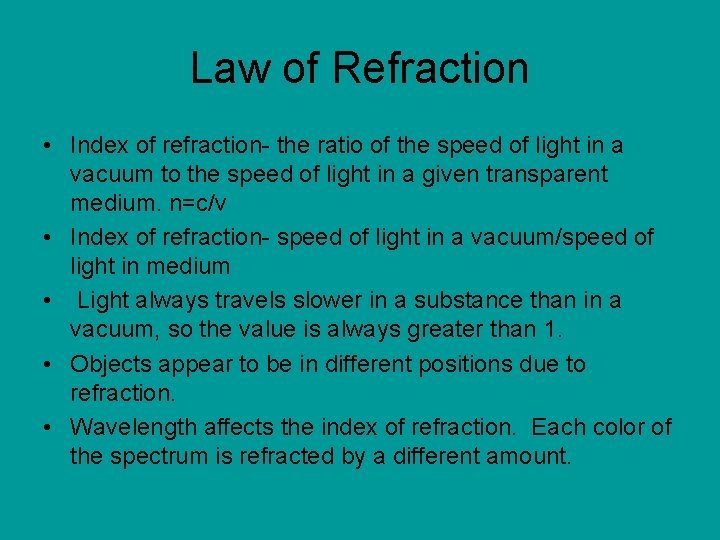Law of Refraction • Index of refraction- the ratio of the speed of light in a vacuum to the speed of light in a given transparent medium. n=c/v • Index of refraction- speed of light in a vacuum/speed of light in medium • Light always travels slower in a substance than in a vacuum, so the value is always greater than 1. • Objects appear to be in different positions due to refraction. • Wavelength affects the index of refraction. Each color of the spectrum is refracted by a different amount.Snell’s Law determines the angle of refraction: • ni sin θi =nr sin θr • The index of refraction of the first medium x sin θ incidence = index of refraction of second medium x sin θ refraction.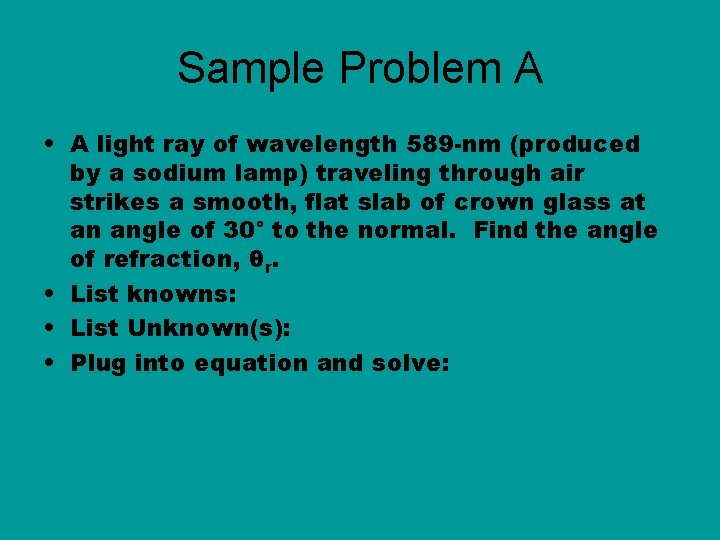Sample Problem A • A light ray of wavelength 589 -nm (produced by a sodium lamp) traveling through air strikes a smooth, flat slab of crown glass at an angle of 30° to the normal. Find the angle of refraction, θr. • List knowns: • List Unknown(s): • Plug into equation and solve: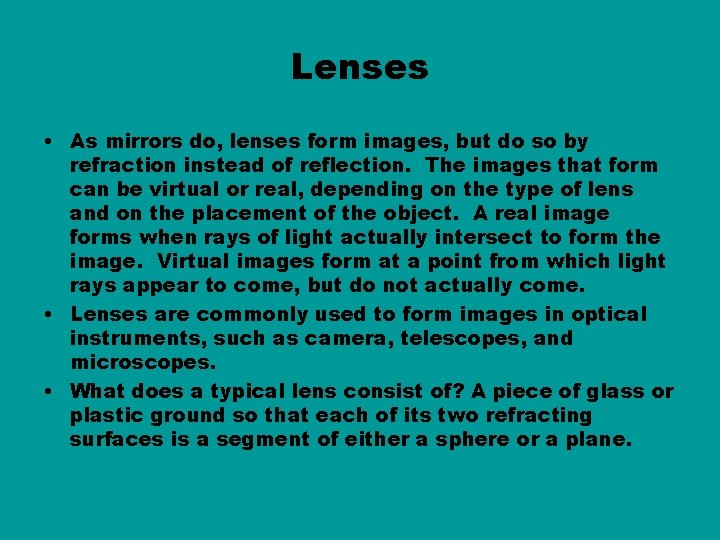Lenses • As mirrors do, lenses form images, but do so by refraction instead of reflection. The images that form can be virtual or real, depending on the type of lens and on the placement of the object. A real image forms when rays of light actually intersect to form the image. Virtual images form at a point from which light rays appear to come, but do not actually come. • Lenses are commonly used to form images in optical instruments, such as camera, telescopes, and microscopes. • What does a typical lens consist of? A piece of glass or plastic ground so that each of its two refracting surfaces is a segment of either a sphere or a plane.Diverging and converging lenses: • Converging lens-lens that is thicker at the middle than the rim. They can produce real or virtual images of real objects, but diverging lenses produce virtual images from real objects. • Diverging lens-lens that is thinner at the middle than it is at the rim.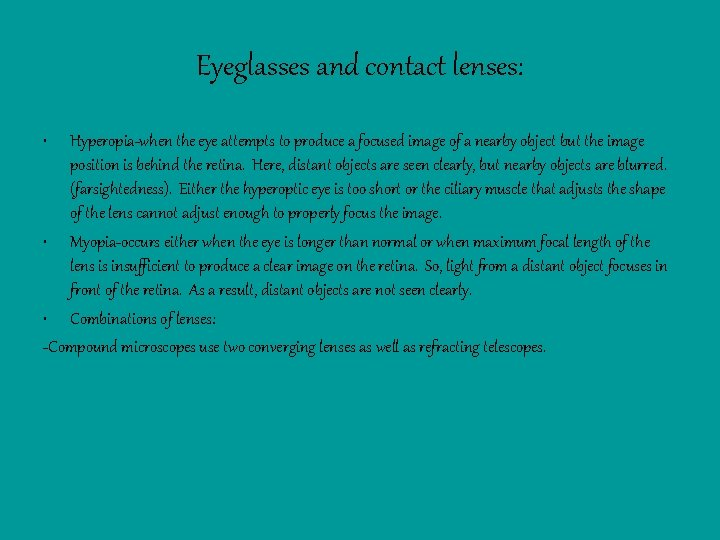Eyeglasses and contact lenses: • Hyperopia-when the eye attempts to produce a focused image of a nearby object but the image position is behind the retina. Here, distant objects are seen clearly, but nearby objects are blurred. (farsightedness). Either the hyperoptic eye is too short or the ciliary muscle that adjusts the shape of the lens cannot adjust enough to properly focus the image. • Myopia-occurs either when the eye is longer than normal or when maximum focal length of the lens is insufficient to produce a clear image on the retina. So, light from a distant object focuses in front of the retina. As a result, distant objects are not seen clearly. • Combinations of lenses: -Compound microscopes use two converging lenses as well as refracting telescopes.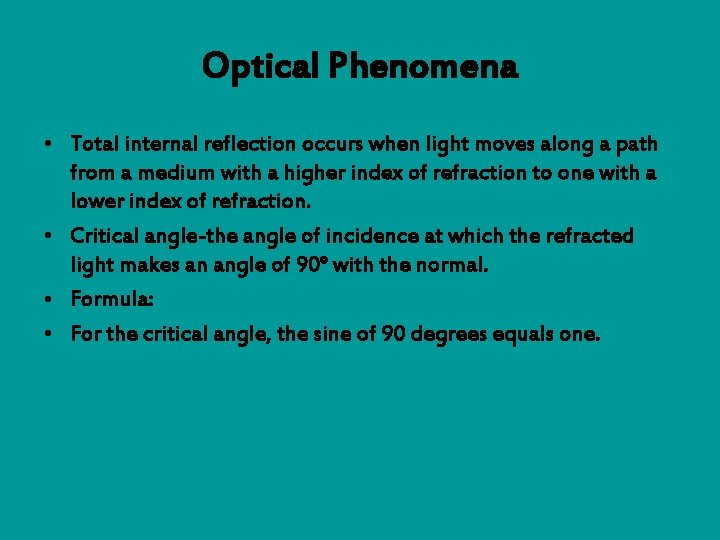Optical Phenomena • Total internal reflection occurs when light moves along a path from a medium with a higher index of refraction to one with a lower index of refraction. • Critical angle-the angle of incidence at which the refracted light makes an angle of 90° with the normal. • Formula: • For the critical angle, the sine of 90 degrees equals one.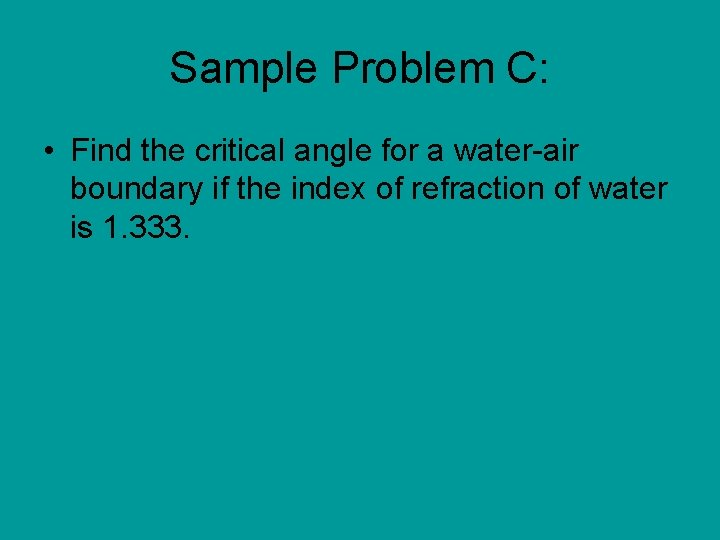Sample Problem C: • Find the critical angle for a water-air boundary if the index of refraction of water is 1. 333.Optical Phenomena: • Refracted light produces mirages. • White light passed through a prism produces a visible spectrum. • Rainbows are created by dispersion of light in water droplets.